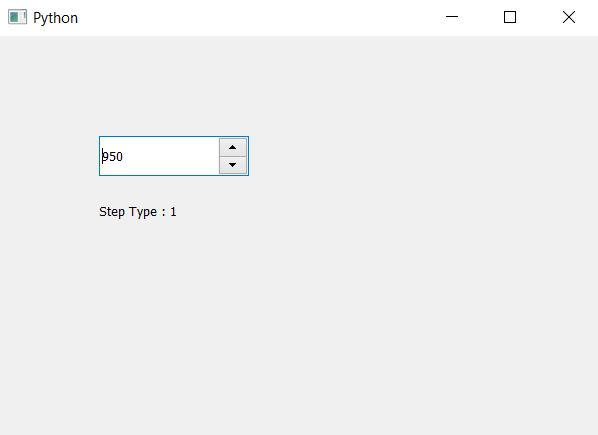Skip to content
Related Articles
PyQt5 QSpinBox – Accessing step type
• Last Updated : 06 May, 2020

In this article we will see how we can access the step type of the spin box, there are two types of step types i.e default one which increment value normally and other is adaptive decimal.

In order to do this we will use `stepType` method

Note : This feature was introduced in Qt 5.12. so lower versions don’t have this feature.

Syntax : spin_box.stepType()

Argument : It takes no argument

Return : It returns QAbstractionSpinBox object but when it printed it shows value 0 or 1

Steps for implementation :
1. Create a spin box
2. Set step type to it with the help of setStepStype method
3. Create label to show the step type
4. Get the step type with the help of stepType method
5. Show type through label

Below is the implementation

 `# importing libraries``from` `PyQt5.QtWidgets ``import` `*` `from` `PyQt5 ``import` `QtCore, QtGui``from` `PyQt5.QtGui ``import` `*` `from` `PyQt5.QtCore ``import` `*` `import` `sys`` ` `class` `Window(QMainWindow):`` ` `    ``def` `__init__(``self``):``        ``super``().__init__()`` ` `        ``# setting title``        ``self``.setWindowTitle(``"Python "``)`` ` `        ``# setting geometry``        ``self``.setGeometry(``100``, ``100``, ``600``, ``400``)`` ` `        ``# calling method``        ``self``.UiComponents()`` ` `        ``# showing all the widgets``        ``self``.show()`` ` `    ``# method for widgets``    ``def` `UiComponents(``self``):`` ` `        ``# creating spin box``        ``self``.spin ``=` `QSpinBox(``self``)`` ` `        ``# setting geometry to spin box``        ``self``.spin.setGeometry(``100``, ``100``, ``150``, ``40``)`` ` `        ``# setting range``        ``self``.spin.setRange(``0``, ``10000``)`` ` `        ``# setting value``        ``self``.spin.setValue(``950``)`` ` `        ``# setting step type``        ``self``.spin.setStepType(QAbstractSpinBox.AdaptiveDecimalStepType)`` ` `        ``# creating label``        ``label ``=` `QLabel(``self``)`` ` `        ``# setting geometry to the label``        ``label.setGeometry(``100``, ``160``, ``200``, ``30``)`` ` `        ``# getting single step size``        ``step_type ``=` `self``.spin.stepType()`` ` `        ``# setting text to the label``        ``label.setText(``"Step Type : "` `+` `str``(step_type))`` ` `# create pyqt5 app``App ``=` `QApplication(sys.argv)`` ` `# create the instance of our Window``window ``=` `Window()`` ` `# start the app``sys.exit(App.``exec``())`

Output :Attention geek! Strengthen your foundations with the Python Programming Foundation Course and learn the basics.

To begin with, your interview preparations Enhance your Data Structures concepts with the Python DS Course. And to begin with your Machine Learning Journey, join the Machine Learning – Basic Level Course

My Personal Notes arrow_drop_up